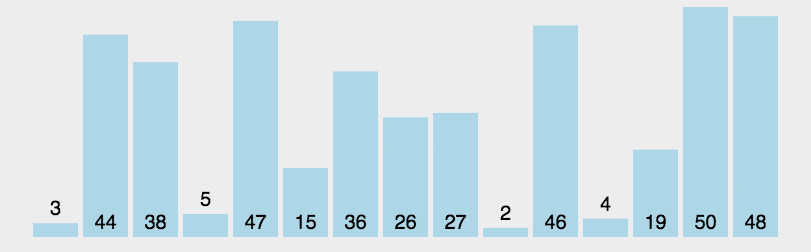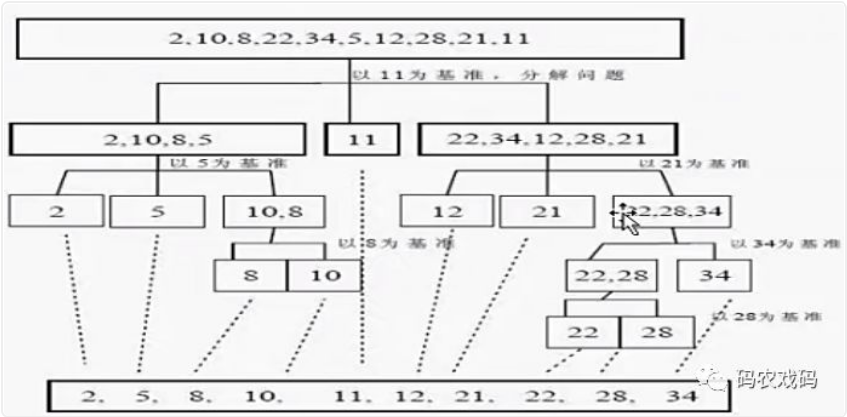# 排序算法之快速排序

+关注继续查看

## 快速排序（Quick Sort）

### 3.动图演示### 4.代码实现

public static void main(String[] args) {
//初始化数组
int[] arr = {5, 3, 1, 4, 2, 7, 8, 6, 10, 9};
quickSort(arr,0,arr.length -1);
System.out.println(Arrays.toString(arr));
}
private static void quickSort(int[] arr,int l, int r){
if(l >= r){
return;
}
int left = l;
int right = r;
int pivot = arr[left];
while(left < right){

while(left < right && arr[right] >= pivot){
right --;
}
if(left < right){
arr[left] = arr[right];
}
while (left < right && arr[left] <= pivot){
left ++;
}
if (left < right){
arr[right] = arr[left];
}else{
arr[left] = pivot;
}
}
quickSort(arr,l,right -1);
quickSort(arr,right + 1,r);
}

public static void main(String[] args) {
//初始化数组
int[] arr = {5, 3, 1, 4, 2, 7, 8, 6, 10, 9};
quickSort(arr, 0, arr.length - 1);
System.out.println(Arrays.toString(arr));
}
private static void quickSort(int[] arr, int l, int r) {
if (l >= r) {
return;
}
int left = l; // 0
int right = r; // 9
int pivot = arr[left]; // 5
while (left < right) {
/**
*  第一次循环：0 < 9 && arr[right] (9)   9 >= 5  条件满足  9 --  = 8
*  第二次循环：0 < 8 && arr[right] (8)   10 >= 5 条件满足  8 --  = 7
*  第三次循环：0 < 7 && arr[right] (7)   6 >= 5  条件满足  7 --  = 6
*  第四次循环：0 < 6 && arr[right] (6)   8 >= 5  条件满足  6 --  = 5
*  第五次循环：0 < 5 && arr[right] (5)   7 >= 5  条件满足  5 --  = 4
*  第六次循环：0 < 4 && arr[right] (4)   2 >= 5  条件不满足  此时 left = 0; right = 4
*/
while (left < right && arr[right] >= pivot) {
right--;
}
/**
*  0 < 4
*/
if (left < right) {
/**
*  arr[left] = 5
*  arr[right] = 2
*  执行之后，数组变成如下：
*  {2, 3, 1, 4, 2, 7, 8, 6, 10, 9};
*/
arr[left] = arr[right];
}
/**
*  第一次循环：0 < 4 && arr[left] (2) <= 5 条件满足  0 ++  = 1
*  第二次循环：1 < 4 && arr[left] (3) <= 5 条件满足  1 ++  = 2
*  第三次循环：2 < 4 && arr[left] (1) <= 5 条件满足  2 ++  = 3
*  第四次循环：3 < 4 && arr[left] (4) <= 5 条件满足  3 ++  = 4
*  第四次循环：4 < 4 条件不满足，此时 left = 4; right = 4
*/
while (left < right && arr[left] <= pivot) {
left++;
}
/**
*  4 < 4 不满足条件进入else
*/
if (left < right) {
arr[right] = arr[left];
} else {
/**
* arr[left] = 2;
* pivot = 5;
* arr[left] = 5;
* 此时数组变成如下：
* {2, 3, 1, 4, 5, 7, 8, 6, 10, 9};
*/
arr[left] = pivot;
}
}
/**
* l = 0
* right = 4
* {2, 3, 1, 4};
* quickSort(arr,0,4-1);
* 排序基准左侧的数
*/
quickSort(arr, l, right - 1);
/**
* right = 4
* r = 9
* {7, 8, 6, 10, 9};
* quickSort(arr,4 + 1,9);
* 排序基准右侧的数
*/
quickSort(arr, right + 1, r);
}



### 5.输出结果

[1, 2, 3, 4, 5, 6, 7, 8, 9, 10]8 023 015 0345 022 032 035 0747 01200 0+关注
60

0